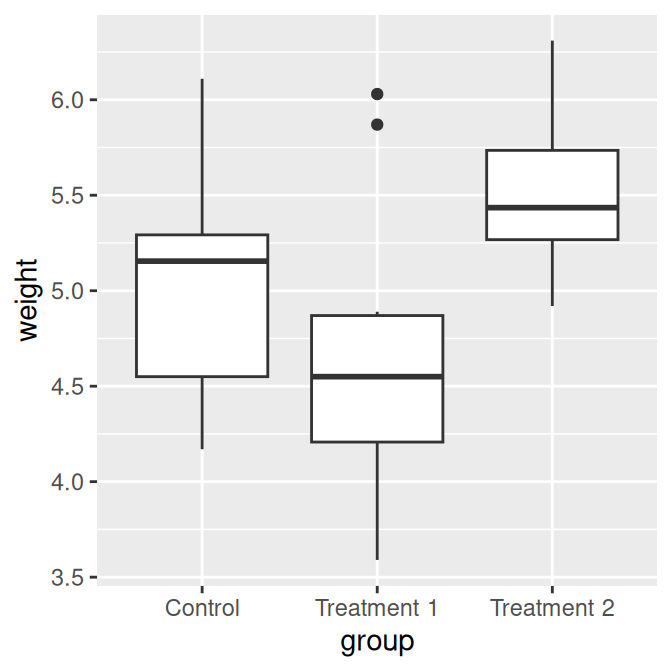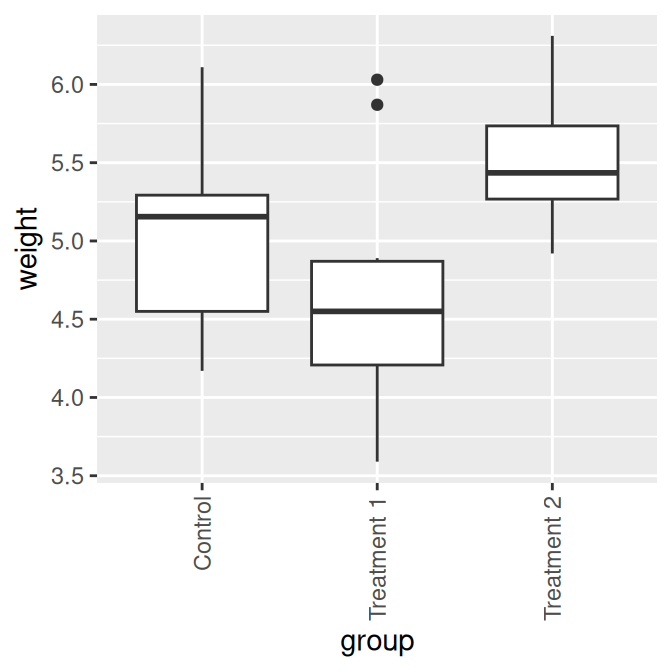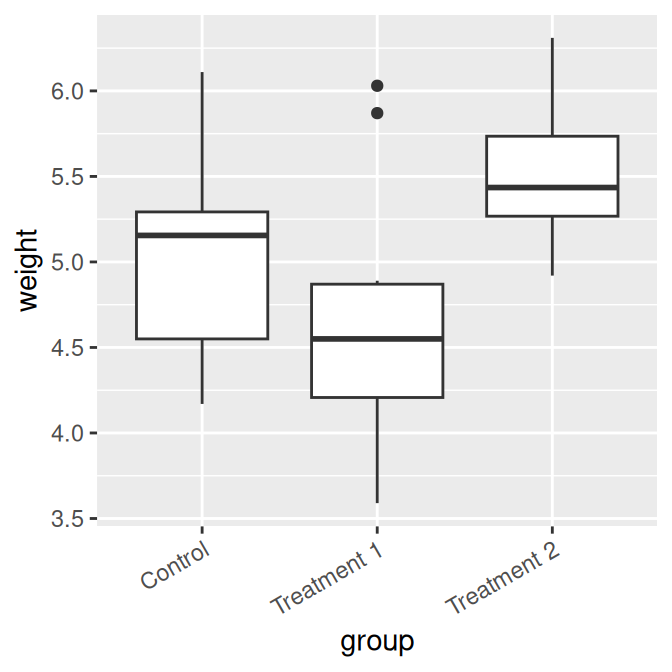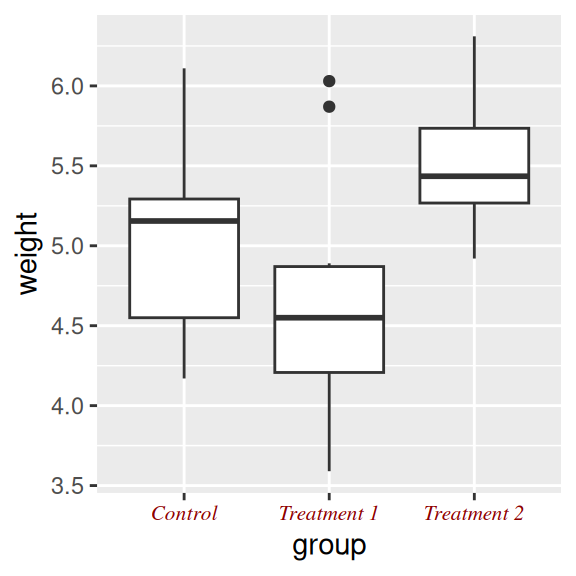## 8.9 Changing the Appearance of Tick Labels

### 8.9.1 Problem

You want to change the appearance of tick labels.

### 8.9.2 Solution

In Figure 8.17 (left), we’ve manually set the labels to be long-long enough that they overlap:

``````pg_plot <- ggplot(PlantGrowth, aes(x = group, y = weight)) +
geom_boxplot() +
scale_x_discrete(
breaks = c("ctrl", "trt1", "trt2"),
labels = c("Control", "Treatment 1", "Treatment 2")
)

pg_plot``````

To rotate the text 90 degrees counterclockwise (Figure 8.17, middle), use:

``````pg_plot +
theme(axis.text.x = element_text(angle = 90, hjust = 1, vjust = .5))``````

Rotating the text 30 degrees (Figure 8.17, right) uses less vertical space and makes the labels easier to read without tilting your head:

``````pg_plot +
theme(axis.text.x = element_text(angle = 30, hjust = 1, vjust = 1))``````Figure 8.17: X-axis tick labels rotated 0 (left), 90 (middle), and 30 degrees (right)

The `hjust` and `vjust` settings specify the horizontal alignment (left/center/right) and vertical alignment (top/middle/bottom).

### 8.9.3 Discussion

Besides rotation, other text properties, such as size, style (bold/italic/normal), and the font family (such as Times or Helvetica) can be set with `element_text()`, as shown in Figure 8.18:

``````pg_plot +
theme(
axis.text.x = element_text(family = "Times", face = "italic",
colour = "darkred", size = rel(0.9))
)``````Figure 8.18: X-axis tick labels with manually specified appearance

In this example, the size is set to `rel(0.9)`, which means that it is 0.9 times the size of the base font size for the theme.

These commands control the appearance of only the tick labels, on only one axis. They don’t affect the other axis, the axis label, the overall title, or the legend. To control all of these at once, you can use the theming system, as discussed in Recipe 9.3.